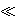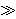### Carsten Elsner, Takao Komatsu and Iekata Shiokawa

FHDW, Fachhochschule für die Wirtschaft, University of Applied Sciences, Freundallee 15, D-30173 Hannover, Germany
e-mail: carsten.elsner@fhdw.de

Graduate School of Science and Technology, Hirosaki University, Hirosaki, 036-8561 Japan
e-mail: komatsu@cc.hirosaki-u.ac.jp

Department of Mathematics, Keio University, Yokohama, 223-8522 Japan
e-mail: shiokawa@math.keio.ac.jp

Abstract.   We compute upper and lower bounds for the approximation of certain values ξ of hyperbolic and trigonometric functions by rationals x/y such that x, y satisfy Diophantine equations. We show that there are infinitely many coprime integers x, y such that

|y ξ - x|(log log y)/(log y)

and a Diophantine equation holds simultaneously relating x, y and some integer z. Conversely, all positive integers x, y with yc0 solving the Diophantine equation satisfy

|y ξ - x|(log log y)/(log y)

Moreover, we approximate sin(πα) and cos(πα) by rationals in connection with solutions of a quadratic Diophantine equation when tan(πα/2) is a Liouville number.

2000 Mathematics Subject Classification.   11D09, 11D25, 11J04, 11J70.

Key words and phrases.   Diophantine approximation, Diophantine equations, trigonometric and hyperbolic functions.

Full text (PDF) (free access)

DOI: 10.3336/gm.44.2.02

References:

1. E. B. Burger and A. M. Pillai, On Diophantine approximation along algebraic curves, Proc. Amer. Math. Soc. 136 (2008), 11-19.
MathSciNet     CrossRef

2. H. Cohen, Number Theory, vol.I: Tools and Diophantine Equations, Springer, 2007.
MathSciNet

3. H. Cohen, Number Theory, vol.II: Analytic and Modern Tools, Springer, 2007.
MathSciNet

4. L. E. Dickson, History of the theory of numbers, vol.2, Dover Publications, 2005.

5. C. Elsner, On arithmetic properties of the convergents of Euler's number, Colloq. Math. 79 (1999), 133-145.
MathSciNet

6. C. Elsner, On rational approximations by Pythagorean numbers, Fibonacci Quart. 41 (2003), 98-104.
MathSciNet

7. C. Elsner, T. Komatsu, and I. Shiokawa, Approximation of values of hypergeometric functions by restricted rationals, J. Théorie des Nombres de Bordeaux 19 (2007), 387-399.
MathSciNet

8. C. Elsner and T. Komatsu, A recurrence formula for leaping convergents of non-regular continued fractions, Linear Algebra Appl. 428 (2008), 824-833.
MathSciNet     CrossRef

9. C. Georgikopoulos, Rational integral solutions of the equations x3+4y3 = z2 and x3+2y3 = z2, Bull. Soc. Math. Gréce 24 (1948), 13-19.
MathSciNet

10. G. H. Hardy and E. M. Wright, An introduction to the theory of numbers, fifth edition, Clarendon Press, Oxford, 1979.
MathSciNet

11. T. Komatsu, Arithmetical properties of the leaping convergents of e1/s, Tokyo J. Math. 27 (2004), 1-12.
MathSciNet     CrossRef

12. L. J. Mordell, Diophantine equations, Academic Press, London and New York, 1969.
MathSciNet

13. O. Perron, Die Lehre von den Kettenbrüchen, Chelsea, New York, 1950.